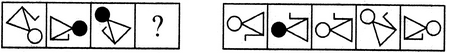# Non Verbal Reasoning - Analogy - Discussion

Discussion Forum : Analogy - Section 1 (Q.No. 12)
Directions to Solve

Each of the following questions consists of two sets of figures. Figures A, B, C and D constitute the Problem Set while figures 1, 2, 3, 4 and 5 constitute the Answer Set. There is a definite relationship between figures A and B. Establish a similar relationship between figures C and D by selecting a suitable figure from the Answer Set that would replace the question mark (?) in fig. (D).

12.

Select a suitable figure from the Answer Figures that would replace the question mark (?).(A)     (B)      (C)     (D)                  (1)      (2)      (3)      (4)      (5)
1
2
3
4
5
Explanation:
The figure rotates 45oACW; the circle changes colour (turns black if initially white and vice-versa). The 'L'-shaped element shifts to the other side of the main figure.
Discussion:
2 comments Page 1 of 1.

Andy said:   2 years ago
Anyone explain the answer in detail.